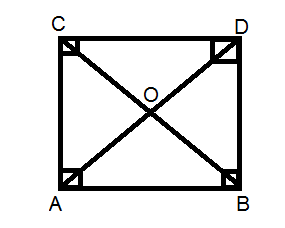Home/Class 8/Maths/

In a square $$ABCD$$, the diagonals meet at point O. The $$\Delta AOB$$ is （ ）
A. Isosceles triangle.
B. Equilateral triangle.
C. Isosceles triangle but not right triangle.
D. Scalene right triangle.Speed
00:00
03:49## QuestionMathsClass 8

In a square $$ABCD$$, the diagonals meet at point O. The $$\Delta AOB$$ is （ ）
A. Isosceles triangle.
B. Equilateral triangle.
C. Isosceles triangle but not right triangle.
D. Scalene right triangle.

A
4.64.6## SolutionThe diagonal of a square bisects each other at $$90^{\circ}$$.
$$\therefore AO=BO$$.
Hence, $$\Delta AOB$$ is a isosceles triangle.